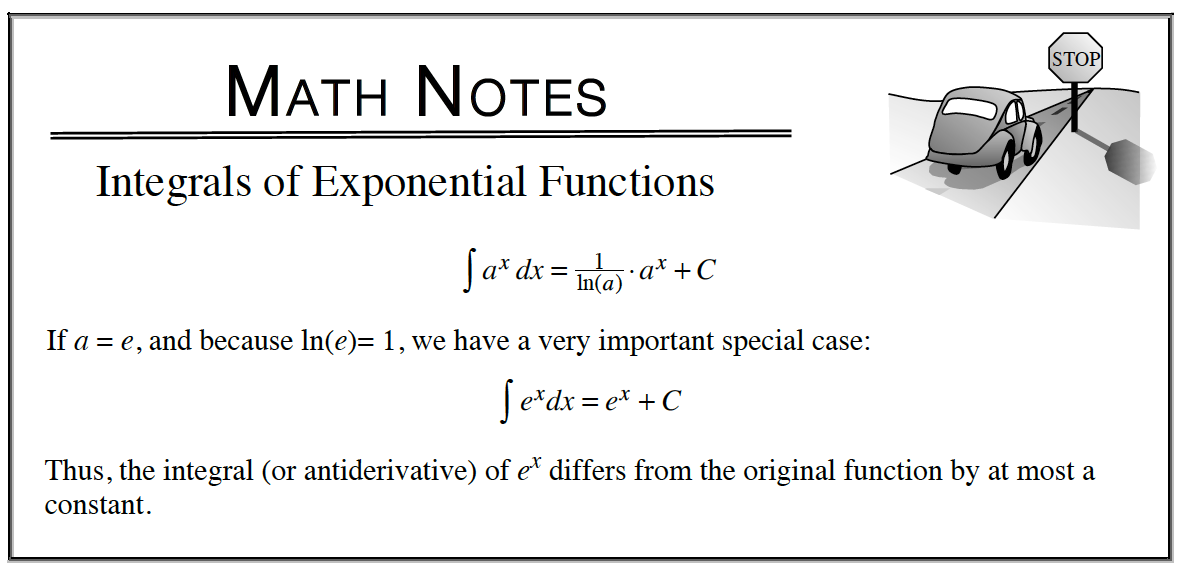Home > APCALC > Chapter 7 > Lesson 7.1.1 > Problem7-7

7-7.

Integrate. Homework Help ✎1. $\int e ^ { 3 x } d x$

If $u= 3x$, what is $du$?

1. $\int \operatorname { sin } ( 3 x ) d x$

What function has a derivative of $\sin(x)$? How can you manipulate this function so it would have a derivative of $\sin(3x)$?

1. $\int [ ( 3 x + 1 ) ^ { 3 } + 3 x + 3 ] d x$

Don't worry if you cannot figure out the antiderivative of $(3x + 1)^3$, you could always expand it first and undo the Power Rule.